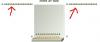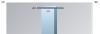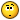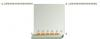Recommended Posts

I have a problem with my Сharts in IE9. It works fine in FireFox, Chrome and IE10/11.

I have those parameters in my graph:

showValues="1" showLegend="1" rotateNames="1" formatNumberScale="1" decimals="1" decimalPrecision="1" limitsDecimalPrecision="0" divLineDecimalPrecision="1" formatNumber="1" chartTopMargin="15" numDivLines='0' yAxisMaxValue='1'

FusionCharts version is 3.6.0.

ThanksEdited by wise

Share on other sites

Hi Moonmi,

I cannot reproduce this issue on the fiddle. It looks like it depends on the response time.

The issue is presented only when I tried to see the report from a server but locally it works fine.

When I debug the code and stop on a breakpoint a few seconds then I can see the issue on my local pc. Its not the issue without stopping on a breakpoint.

Maybe its something related to caching?Edited by wise

Share on other sites

Hi,

If possible can you share with the Live URL of the issue so that we can help you out further?

Awaiting response.

Share on other sites

I downgraded FusionCharts to version 3.2.3-sr3.5347 and fixed the issue with this line:

FusionCharts.setCurrentRenderer('flash');

We have a licence for version 3.6 and this doesnt work for it.

Unfortunately I cannot share the url.

Share on other sites

Hi,

The current version has deprecated the flash component and does not provide support too.

The updated version of FusionCharts is totally JavaScript. Without replicating the issue we cannot comment on this issue.

If possible LiveURL can be of help.

Share on other sites

I create a chart like this:

var dbChart = new FusionCharts({type : "MSColumn3DLineDY", id : "homepeoplexml", width :"450", height:"300", debugMode :"1", registerWithJS :"1"});

dbChart.setXMLUrl("xml.action?vars.............");

dbChart.render("dbchart");

xml.action returns xml:

<?xml version="1.0" encoding="UTF-8"?>

<graph PYAxisName="No." caption="Ratings" SYAxisName="Rating" shownames="1" showvalues="1" showLegend="0" rotateNames="1" formatNumberScale="1" decimals="1"  decimalPrecision="1" limitsDecimalPrecision="0" divLineDecimalPrecision="1" formatNumber="1" palette="4"  numDivLines='0' yAxisMaxValue='1'>
<categories>

<category name="text" hoverText="text"/>
</categories>
<dataset seriesname="No." showValues="1" decimals="1" decimalPrecision="1" parentYAxis="P">

<set value="15" />
</dataset>
<dataset seriesname="Rating" showValues="1" decimals="1" decimalPrecision="1" color="FF8000" anchorBorderColor="FF8000" parentYAxis="S" lineThickness="4">

<set value="42.33" />
</dataset>
</graph>

And I have this picture:Edited by wise

Share on other sites

It looks like I have this issue only with 'MSColumn3DLineDY' type. 'Pie3D', 'Column3D', and 'Area2D' work fineTried to use 'charts' tag instead of 'graph', to set decimals="0" - the same issue. With decimals="0" I have this picture:Share on other sites

xml:

<?xml version="1.0" encoding="UTF-8"?>
<graph PYAxisName="No." caption="Statistics" SYAxisName="Rating" shownames="1" showValues="1" showLegend="1" rotateNames="1" formatNumberScale="1" decimals="1" decimalPrecision="1" limitsDecimalPrecision="0" divLineDecimalPrecision="1" formatNumber="1" chartTopMargin="15" numDivLines='0' yAxisMaxValue='1'>

<categories>

<category name="New" hoverText="Text"/>
<category name="Used" hoverText="Text"/>
<category name="Collect" hoverText="Text"/>
<category name="Service" hoverText="Text"/>
<category name="Parts" hoverText="Text"/>
<category name="Demo" hoverText="Text"/>
</categories>
<dataset seriesname="No." showValues="1" decimals="1" decimalPrecision="1" color="19BE07" parentYAxis="P">

<set value=""/>
<set value=""/>
<set value=""/>
<set value=""/>
<set value=""/>
<set value=""/>
</dataset>
<dataset seriesname="Rating" showValues="1" decimals="1" decimalPrecision="1" color="FF8000" anchorBorderColor="FF8000" parentYAxis="S" lineThickness="4">

<set value="0.0"/>
<set value="0.0"/>
<set value="0.0"/>
<set value="0.0"/>
<set value="0.0"/>
<set value="0.0"/>
</dataset>
</graph>

Edited by wise

Share on other sites

Works fine if I put xml data to the chart by setXMLData function. So the issue is in setXMLUrl function if it takes time to load the data.

Share on other sites

Hi,

Refer this sample JSFiddle: http://jsfiddle.net/fusioncharts/MSDV4/

Hope this helps.

Share on other sites

Hi,

Can you please share the scaled down sample so that we can suggest you more?

Awaiting response.